MORE IN Engineering Mechanics (EM)
SPPU First Year Engineering (Semester 2)
Engineering Mechanics (EM)
October 2012
Total marks: --
Total time: --
INSTRUCTIONS
(1) Assume appropriate data and state your reasons
(2) Marks are given to the right of every question
(3) Draw neat diagrams wherever necessary

Answer any one question from Q1 & Q2
1 (a) Find the magnitude of the resultant and its location of the following forces acting at a point O as shown in Fig. 1.a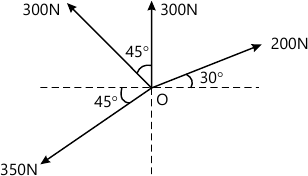6 M
1 (b) A Particle starts with an initial velocity of 2.5 m/s and uniformly accelerates at the rate 0.5 m/s2. Determine the displacement in 2 s, time required to attain the velocity of 7.5 m/s and the distance travelled when it attain a velocity of 7.5 m/s.
6 M

2 (a) Determine the position of centroid of the shaded area as shown in Fig. 2a with respect to origin O.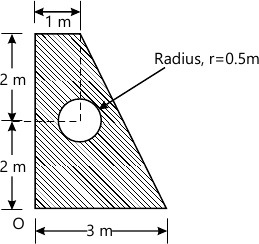6 M
2 (b) Two weights 100 N and 30 N are connected by a string and move along a rough horizontal plane under the action of force 50 N applied to the first weight 100 N as shown in Fig. 2 b. The coefficient of friction between the sliding surfaces of the weights and plane is 0.25. Determine the acceleration of weights and the tension in the string using Newton's second law.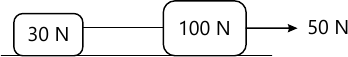6 M

Answer any one question from Q3 & Q4
3 (a) A square foundation supports four loads as shown in Fig. 3 a. Determine magnitude, direction and point of application of resultant of four forces.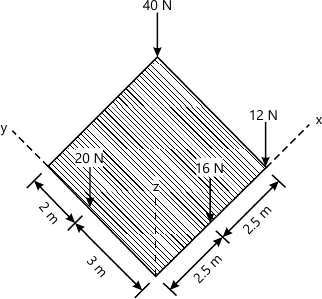6 M
3 (b) Two sphere P and Q each of weight 50 N and a radius of 100 mm rest in horizontal channel of width 360 mm as shown in Fig. 3 b. Determine the reaction at the point of contact A, B and C.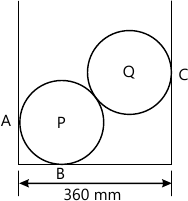7 M
3 (c) A particle is projected at an angle of 30° to the horizontal with a velocity of 100 m/s. Determine the range of radius of curvature of the path followed by the particle.
6 M

4 (a) Three loads are applied as shown in Fig. 4 a. to a light beam supported by cables attached at B and C. Neglecting the weight of the beam, determine the range of values of Q for which neither cable becomes slack when P = 0.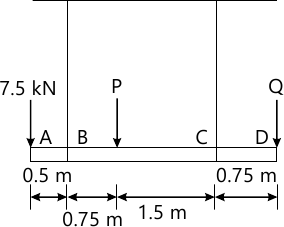7 M
4 (b) A 200 kg cylinder is hung by means of two cables AB and AC, which isattached to the top of vertical wall. A horizontal force P perpendicular to thewall holds the cylinder in the position shown in Fig. 4 b. Determine the magnitudeof P and the tension in each cable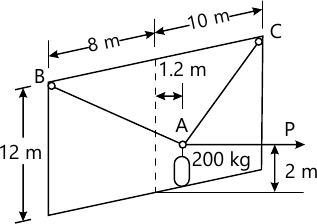6 M
4 (c) A 150 kg car enters a curved portion of the road of radius 200 m travelling at a constant speed of 36 km/h. Determine the normal and tangential component of force at curved portion.
6 M

Answer any one question from Q5 & Q6
5 (a) A plane truss is loaded and supported as shown in Fig.5 a. Determine the magnitude and nature of forces in all the members.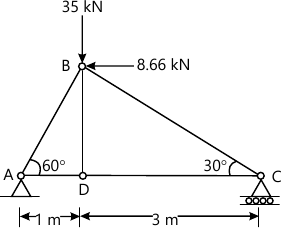6 M
5 (b) A body of weight 300 N is kept on a rough horizontal plane and a force P is applied to just move the body horizontally as shown in Fig. 5 b. Find the magnitude of force P required if coefficient of static friction is ?s=0.4.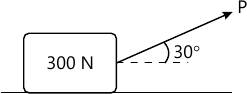7 M
5 (c) A car of mass 1500 kg is moving down a hill having a slope of 15° to the horizontal. At the time, when the car is moving at a speed of 10 m/s, the driver applies the brakes. Calculate the average force applied parallel to the hill slope that will stop the car in a distance of 30 m. Use work energy principle.
:IMAGE-
6 M

6 (a) Determine the reactions at A, D and tension in BC of the rope ABCD loaded and supported as shown in Fig.6 a.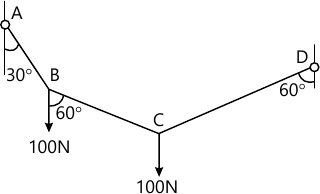6 M
6 (b) A 100 N ladder AB of length 6 m rest against a vertical wall and horizontal floor as shown in Fig. 6 b. Determine the slope of the ladder with vertical to maintain equilibrium if the coefficient of static friction at all contact surface is ?s=0.25.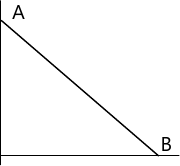7 M
6 (c) Determine the velocities of the two balls shown in Fig. 6 C after impact. Take weight of ball A is 20 N, weight of ball B is 10 N and coefficient of restitution is 0.6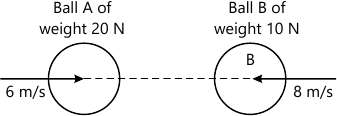6 M

More question papers from Engineering Mechanics (EM)